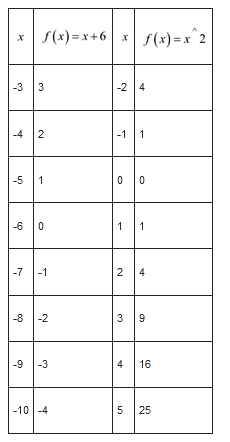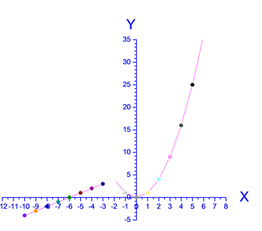# The given piecewise function is f ( x ) = { x + 6 , if x &lt; − 2 x 2 , if x ≥ − 2### Precalculus: Mathematics for Calcu...

6th Edition
Stewart + 5 others
Publisher: Cengage Learning
ISBN: 9780840068071### Precalculus: Mathematics for Calcu...

6th Edition
Stewart + 5 others
Publisher: Cengage Learning
ISBN: 9780840068071

#### Solutions

Chapter 2, Problem 39RE
To determine

## The given piecewise function is f(x)={x+6,  if x<−2x2,      if x≥−2

Expert Solution

Evaluate the function for x , make a table of values, and plot the graph.

### Explanation of Solution

Given information: The given piecewise function is f(x)={x+6,  if x<2x2,      if x2

Calculation:

Give some values and draw the table below:Thus, the graph as shown below:### Have a homework question?

Subscribe to bartleby learn! Ask subject matter experts 30 homework questions each month. Plus, you’ll have access to millions of step-by-step textbook answers!